Help & Support
Send FeedbackMath Equations
Convert Latex, AsciiMath and MathML equations to an image.
698,687 users
Works with
Install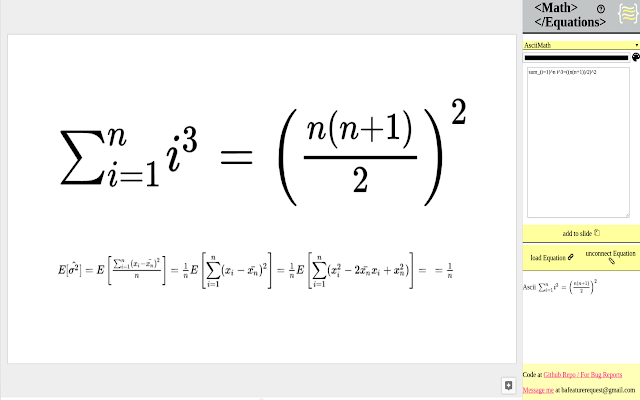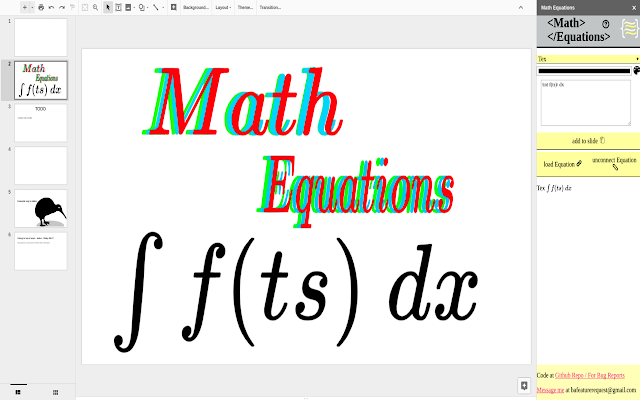Overview
```Math Equations allows you to take your typeset languages and convert them to images to use inside of your slideshow.  Other features include the ability to reload a equation image and make changes and re upload to your presentation.

Recent changes added the math equations data into the alt text, which you can see by clicking using control + alt + y.

Supported Languages are
-Latex
-AsciiMath
-MathML

Other Features
-Font Color
-Font Size

If you have any problems just send my a message at
bafeaturerequest@gmail.com
Code can be located at

If this extension is interested you can check out my other extension that allows you to create math equations on any site.

Examples
Tex
--valid Tex equation

x^n + y^n = z^n

Tex type uses inline notation.  Which restricts what you can add in your equation.

Add \[ ]\ will cause a error at the current iteration.
Also many formatting tags and the use of usepackage will cause a error. Some of these feature i will include after i build a separate window to work on larger equations.

MathML
--Valid MathML

<mi>&#x3C0;</mi>
<mo>&#x2062;</mo>
<msup>
<mi>r</mi>
<mn>2</mn>
</msup>

MathML doesn't need to have a [itex] tag to be a valid equation.

AsciiMath
--Valid AsciiMath

sum_(i=1)^n i^3=((n(n+1))/2)^2

```
ReviewsA User of Math Equations
December 8, 2019
Works well, just know that the equation is rendered as an image (perhaps not surprising). Also, most of the negative reviews are from people who don't understand LaTeX. I would ignore those...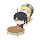A User of Math Equations
November 29, 2019
It's the current best thing we have for equations for google slides, but I wish there were a way for the equations to resize based on the inline text size.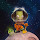A User of Math Equations
November 20, 2019
Works perfectly! You will see many reviews saying that it is difficult to use, but that is not the case IF you understand how to write equations in LaTeX. Contrary to what others have said, you can in fact edit an equation after creating it using the equation linking feature. This add-on is absolutely essential for me! No more taking screenshots of equations and pasting them in with a solid background. I wish I could post images with this review. I'd show you how great it is.A User of Math Equations
October 23, 2019
I don't understand how to use it and there are no instructionsA user of Math Equations
November 10, 2019
well yea cuz u need to learn the code first before you use it. this add-on just lets you turn the code into an image that is much easier to understand and looks so much better than just writing your equation in plain text.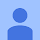Pamela Henderson
October 4, 2019
Too difficult to use.A user of Math Equations
November 20, 2019
I assume you don't know how to use LaTeX? Understanding how LaTeX is used is required for this app.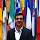A User of Math Equations
October 4, 2019
This is just a picture generator. The equations cannot be edited once created. The equations can also not be generated in line with other text. However, I am even more frustrated that google slides does not have any ability to add equations. Has google stopped developing slides?A user of Math Equations
November 19, 2019
That is not true! You can edit them! Click on the equation you wish to edit, then click the "Connect to equation" button in the Math equation panel.A User of Math Equations
July 29, 2019
few weeks ago the UI is not working anymore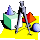A User of Math Equations
May 6, 2019
This is the best equation editor for google slides. Should add support for inline equations though I can see why it is difficult to do as add-on.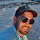A User of Math Equations
April 19, 2019
Very awkward. When the equations use a set of words instead of symbols it becomes useless. The interface is very bad.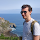A User of Math Equations
April 14, 2019
Nice concept. However, the size setting controls vertical resolution instead of dpi, which somehow varies wildly. This results in some equations (especially long single line ones) looking quite crappy.
1-10 of 26